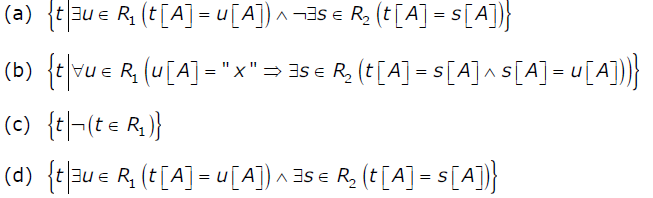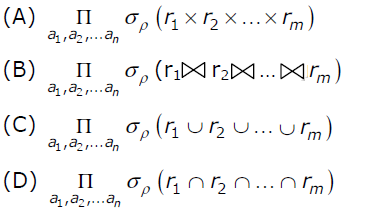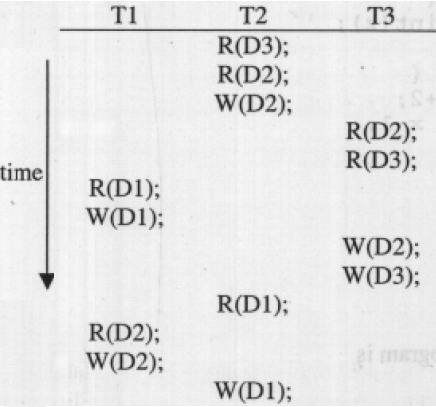81. Suppose a database schedule S involves transactions T1, ....Tn. Construct the precedence graph of S with vertices representing the transactions and edges representing the conflicts. If S is serializable, which one of the following orderings of the vertices of the precedence graph is guaranteed to yield a serial schedule? a. Topological order b. Depth-first order c. Breadth-first order d. Ascending order of transaction indices

 82. Consider the following database schedule with two transactions, T1 and T2.S = r2(X); r1(X); r2(Y); w1(X); r1(Y); w2(X); a1; a2;where ri(Z) denotes a read operation by transaction Ti on a variable Z, wi(Z) denotes a write operation by Ti on a variable Z and ai denotes an abort by transaction Ti . Which one of the following statements about the above schedule is TRUE? a. S is non-recoverable b. S is recoverable, but has a cascading abort c. S does not have a cascading abort d. S is strict

 83. Consider the following three schedules of transactions T1, T2 and T3. [Notation: In the following NYO represents the action Y (R for read, W for write) performed by transac­tion N on object O.](S1) 2RA 2WA 3RC 2WB 3WA 3WC 1RA 1RB 1WA 1WB(S2) 3RC 2RA 2WA 2WB 3WA 1RA 1RB 1WA 1WB 3WC(S3) 2RA 3RC 3WA 2WA 2WB 3WC 1RA 1RB 1WA 1WBWhich of the following statements is TRUE? a. S1, S2 and S3 are all conflict equivalent to each other b. No two of S1, S2 and S3 are conflict equivalent to each other c. S2 is conflict equivalent to S3, but not to S1 d. S1 is conflict equivalent to S2, but not to S3

 84. Which of the following statement is/are incorrect? A: A schedule following strict two phase locking protocol is conflict serializable as well as recoverable.B: Checkpoint in schedules are inserted to ensure recoverability. a. Only 1 b. Only 2 c. Both 1 and 2 d. None

 85. An index is clustered, if a. it is on a set of fields that form a candidate key b. it is on a set of fields that include the primary key c. the data records of the file are organized in the same order as the data entries of the index d. the data records of the file are organized not in the same order as the data entries of the index
 View Answer Report Discuss Too Difficult! Answer: (c).the data records of the file are organized in the same order as the data entries of the index

 86. A clustering index is defined on the fields which are of type a. non-key and ordering b. non-key and non-ordering c. key and ordering d. key and non-ordering

 87. A file is organized so that the ordering of data records is the same as or close to the ordering of data entries in some index. Then that index is called a. Dense b. Sparse c. Clustered d. Unclustered
 88. Which of the following relational calculus expressions is not safe?a. a b. b c. c d. d
 89. Consider the following SQL query:select distinct al, a2,........., anfrom r1, r2,........, rmwhere P For an arbitrary predicate P, this query is equivalent to which of the following relational algebra expressions ?a. A b. B c. C d. D
 90. Consider three data items D1, D2 and D3 and the following execution schedule of transactions T1, T2 and T3. In the diagram, R(D) and W(D) denote the actions reading and writing the data item D respectively. Which of the following statements is correct?a. The schedule is serializable as T2; T3; T1 b. The schedule is serializable as T2; T1; T3 c. The schedule is serializable as T3; T2; T1 d. The schedule is not serializable